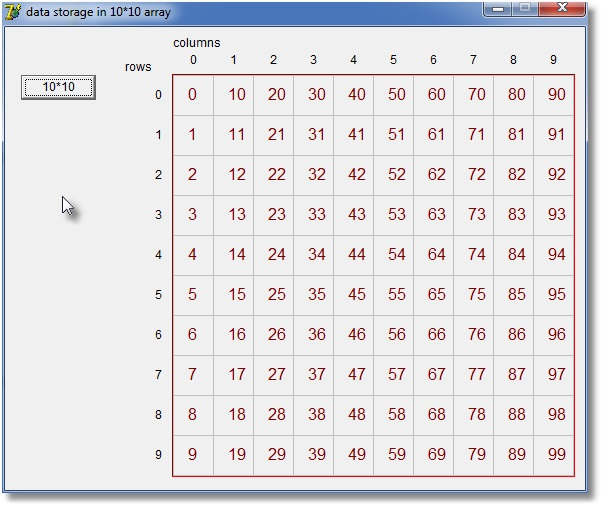Data storage in 2 dimensional arraysComputer memory is a linear list of bytes.
In 2 dimensional arrays, data is stored organised in columns and rows.
We wonder how these columns and rows are positioned in memory.

To investigate this question, we define a 1 dimensional array of 100 bytes.
Also, we define a 2 dimensional array of 10*10 bytes at the same memory locations.

The 1 dimensional array is filled with numbers 0,1,2,... the same data as it's index.
Then the 2 dimensional array contents are read and displayed in a 10* 10 table.

Program

```type TB = array[0..9,0..9] of byte;
PB = ^TB;

var a : array[0..99] of byte;

procedure TForm1.Button1Click(Sender: TObject);
//show position of data in 10*10 array
var i,j : byte;
p : Pb;
begin
p := PB(@a);
for i := 0 to 99 do a[i] := i;
with paintbox1.canvas do
begin
font.Name := 'arial';
font.Height := 18;
font.Color := \$80;
brush.Style := bsClear;
for j := 0 to 9 do
for i := 0 to 9 do
textout(15+i*40,10+j*40,inttostr(p^[i,j]));
end;
end;
```
Below is a snapshot of the resultsBesides pointers, there is another, more simple, way to match the arrays:
```var a : array[0..99] of byte;
b : array[0..9,0..9] of byte absolute a;
```
a and b now occupy the same memory space.

What do we learn from this exercise?

Say we have a 2 dimensional array with cc columns and rr rows: array[0..cc-1,0..rr-1]
Element at column c and row r is adressed as arrayname2 [c,r]

The same element is addressed in a 1 dimensional array as arrayname1[n]

1. converting [c,r] to n:
n := rr*c + r
2.converting n to [c,r]:
c := n div rr;
r := n mod rr;